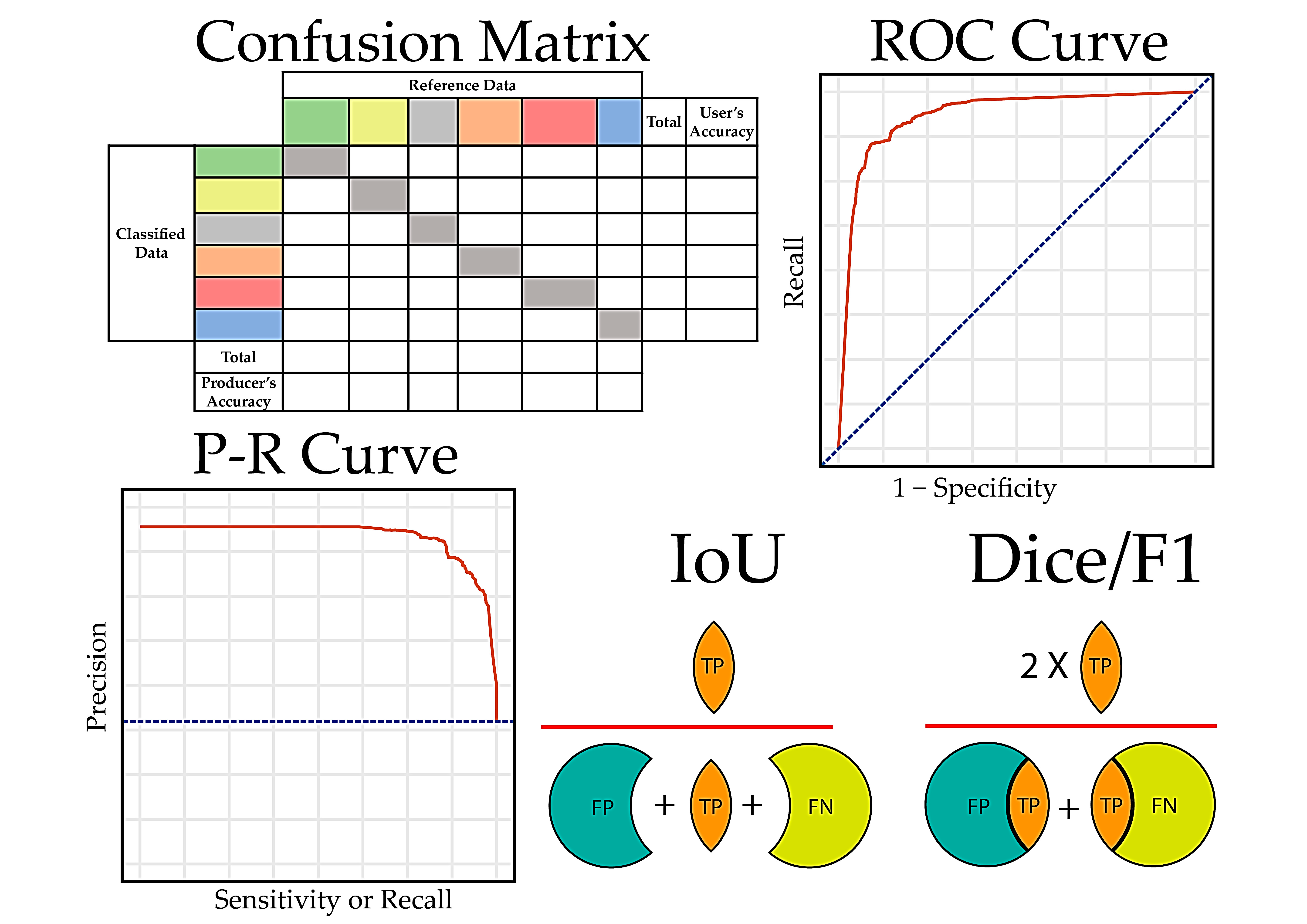# Beyond Classification Accuracy: Exploring More Performance Measures in Machine Learning# Beyond Classification Accuracy: Exploring More Performance Measures in Machine Learning

In the realm of machine learning, classification accuracy is often the first metric we turn to when evaluating the performance of a model. However, relying solely on classification accuracy can be misleading, especially in cases where the classes are imbalanced. This comprehensive guide will delve into why classification accuracy is not always enough and explore other performance measures that can provide a more holistic view of your model’s performance.

## The Limitations of Classification Accuracy

Classification accuracy is the ratio of correct predictions to total predictions made. While it’s a straightforward and intuitive metric, it doesn’t tell the whole story, especially when dealing with imbalanced classes. For instance, if you have a dataset where 95% of the instances belong to Class A and only 5% belong to Class B, a model that always predicts Class A will have an accuracy of 95%. However, this model is not useful as it fails to correctly classify any instances of Class B.

This is known as the Accuracy Paradox. While the model’s accuracy is high, it lacks predictive power, particularly for the minority class. This is why it’s essential to consider other performance measures in addition to classification accuracy.

## The Confusion Matrix

A confusion matrix, also known as a contingency table, provides a more detailed breakdown of a classifier’s performance. It’s a 2×2 table for binary classification problems, with the rows representing the predicted class and the columns representing the actual class. The four cells of the matrix represent:

– True Positives (TP): Instances correctly predicted as positive.
– True Negatives (TN): Instances correctly predicted as negative.
– False Positives (FP): Negative instances incorrectly predicted as positive.
– False Negatives (FN): Positive instances incorrectly predicted as negative.

The confusion matrix provides a clear picture of the model’s performance, highlighting the number of correct and incorrect predictions, and the types of errors made.

## Precision and Recall

Two important metrics derived from the confusion matrix are Precision and Recall.

– Precision (also known as Positive Predictive Value) is the ratio of True Positives to the sum of True Positives and False Positives. It measures the model’s exactness or quality. A high precision indicates a low rate of false-positive errors.

– Recall (also known as Sensitivity or True Positive Rate) is the ratio of True Positives to the sum of True Positives and False Negatives. It measures the model’s completeness or quantity. A high recall indicates a low rate of false-negative errors.

## F1 Score

The F1 Score is the harmonic mean of Precision and Recall, providing a balance between the two. It’s particularly useful in situations where both Precision and Recall are important. A high F1 Score indicates that both the Precision and Recall of the model are high.

## Relevant Prompts for Understanding Performance Measures

To help you get started with understanding these performance measures, here are some prompts that you can use:

1. “What is the Accuracy Paradox in machine learning?”
2. “How does a confusion matrix provide a more detailed view of a model’s performance than classification accuracy?”
3. “What are Precision and Recall, and how are they calculated?”
4. “Why are Precision and Recall important metrics in machine learning?”
5. “What is the F1 Score, and when is it used?”
6. “How can Precision and Recall help in evaluating models on imbalanced datasets?”
7. “What are the limitations of using classification accuracy as the sole performance measure?”
8. “How can the confusion matrix help in understanding the types of errors made by a model?”
9. “What is the relationship between Precision, Recall, and the F1 Score?”
10. “How can these performance measures help in improving the performance of a machine learning model?”

In conclusion, while classification accuracy is a useful starting point, it’s not always sufficient for evaluating the performance of a machine learning model. By understanding and utilizing other performance measures like the confusion matrix, Precision, Recall, and the F1 Score, you can gain a more comprehensive understanding of your model’s performance and make more informed decisions in your machine learning projects.

## Find more … …

Machine Learning for Beginners in Python: F1 Score

Python Data Science – How to check model’s recall score using cross validation in Python

Python Data Science – How to check model’s precision score using cross validation in Python